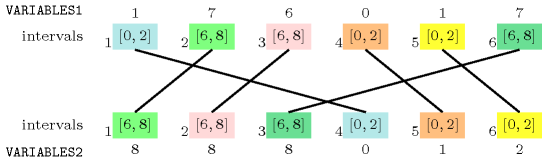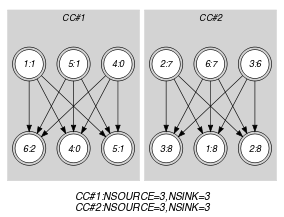## 5.338. same_interval

Origin

Derived from $\mathrm{𝚜𝚊𝚖𝚎}$.

Constraint

$\mathrm{𝚜𝚊𝚖𝚎}_\mathrm{𝚒𝚗𝚝𝚎𝚛𝚟𝚊𝚕}\left(\mathrm{𝚅𝙰𝚁𝙸𝙰𝙱𝙻𝙴𝚂}\mathtt{1},\mathrm{𝚅𝙰𝚁𝙸𝙰𝙱𝙻𝙴𝚂}\mathtt{2},\mathrm{𝚂𝙸𝚉𝙴}_\mathrm{𝙸𝙽𝚃𝙴𝚁𝚅𝙰𝙻}\right)$

Arguments
 $\mathrm{𝚅𝙰𝚁𝙸𝙰𝙱𝙻𝙴𝚂}\mathtt{1}$ $\mathrm{𝚌𝚘𝚕𝚕𝚎𝚌𝚝𝚒𝚘𝚗}\left(\mathrm{𝚟𝚊𝚛}-\mathrm{𝚍𝚟𝚊𝚛}\right)$ $\mathrm{𝚅𝙰𝚁𝙸𝙰𝙱𝙻𝙴𝚂}\mathtt{2}$ $\mathrm{𝚌𝚘𝚕𝚕𝚎𝚌𝚝𝚒𝚘𝚗}\left(\mathrm{𝚟𝚊𝚛}-\mathrm{𝚍𝚟𝚊𝚛}\right)$ $\mathrm{𝚂𝙸𝚉𝙴}_\mathrm{𝙸𝙽𝚃𝙴𝚁𝚅𝙰𝙻}$ $\mathrm{𝚒𝚗𝚝}$
Restrictions
 $|\mathrm{𝚅𝙰𝚁𝙸𝙰𝙱𝙻𝙴𝚂}\mathtt{1}|=|\mathrm{𝚅𝙰𝚁𝙸𝙰𝙱𝙻𝙴𝚂}\mathtt{2}|$ $\mathrm{𝚛𝚎𝚚𝚞𝚒𝚛𝚎𝚍}$$\left(\mathrm{𝚅𝙰𝚁𝙸𝙰𝙱𝙻𝙴𝚂}\mathtt{1},\mathrm{𝚟𝚊𝚛}\right)$ $\mathrm{𝚛𝚎𝚚𝚞𝚒𝚛𝚎𝚍}$$\left(\mathrm{𝚅𝙰𝚁𝙸𝙰𝙱𝙻𝙴𝚂}\mathtt{2},\mathrm{𝚟𝚊𝚛}\right)$ $\mathrm{𝚂𝙸𝚉𝙴}_\mathrm{𝙸𝙽𝚃𝙴𝚁𝚅𝙰𝙻}>0$
Purpose

Let ${N}_{i}$ (respectively ${M}_{i}$) denote the number of variables of the collection $\mathrm{𝚅𝙰𝚁𝙸𝙰𝙱𝙻𝙴𝚂}\mathtt{1}$ (respectively $\mathrm{𝚅𝙰𝚁𝙸𝙰𝙱𝙻𝙴𝚂}\mathtt{2}$) that take a value in the interval $\left[\mathrm{𝚂𝙸𝚉𝙴}_\mathrm{𝙸𝙽𝚃𝙴𝚁𝚅𝙰𝙻}·i,\mathrm{𝚂𝙸𝚉𝙴}_\mathrm{𝙸𝙽𝚃𝙴𝚁𝚅𝙰𝙻}·i+\mathrm{𝚂𝙸𝚉𝙴}_\mathrm{𝙸𝙽𝚃𝙴𝚁𝚅𝙰𝙻}-1$. For all integer $i$ we have ${N}_{i}={M}_{i}$.

Example
$\left(〈1,7,6,0,1,7〉,〈8,8,8,0,1,2〉,3\right)$

In the example, the third argument $\mathrm{𝚂𝙸𝚉𝙴}_\mathrm{𝙸𝙽𝚃𝙴𝚁𝚅𝙰𝙻}=3$ defines the following family of intervals $\left[3·k,3·k+2\right]$, where $k$ is an integer. Consequently the values of the collection $〈1,7,6,0,1,7〉$ are respectively located within intervals $\left[0,2\right]$, $\left[6,8\right]$, $\left[6,8\right]$, $\left[0,2\right]$, $\left[0,2\right]$, $\left[6,8\right]$. Therefore intervals $\left[0,2\right]$ and $\left[6,8\right]$ are respectively used 3 and 3 times. Similarly, the values of the collection $〈8,8,8,0,1,2〉$ are respectively located within intervals $\left[6,8\right]$, $\left[6,8\right]$, $\left[6,8\right]$, $\left[0,2\right]$, $\left[0,2\right]$, $\left[0,2\right]$. As before intervals $\left[0,2\right]$ and $\left[6,8\right]$ are respectively used 3 and 3 times. Consequently the $\mathrm{𝚜𝚊𝚖𝚎}_\mathrm{𝚒𝚗𝚝𝚎𝚛𝚟𝚊𝚕}$ constraint holds. Figure 5.338.1 illustrates this correspondence.

##### Figure 5.338.1. Illustration of the correspondence between the items of the $\mathrm{𝚅𝙰𝚁𝙸𝙰𝙱𝙻𝙴𝚂}\mathtt{1}$ and of the $\mathrm{𝚅𝙰𝚁𝙸𝙰𝙱𝙻𝙴𝚂}\mathtt{2}$ collections of the Example slotTypical
 $|\mathrm{𝚅𝙰𝚁𝙸𝙰𝙱𝙻𝙴𝚂}\mathtt{1}|>1$ $\mathrm{𝚛𝚊𝚗𝚐𝚎}$$\left(\mathrm{𝚅𝙰𝚁𝙸𝙰𝙱𝙻𝙴𝚂}\mathtt{1}.\mathrm{𝚟𝚊𝚛}\right)>1$ $\mathrm{𝚛𝚊𝚗𝚐𝚎}$$\left(\mathrm{𝚅𝙰𝚁𝙸𝙰𝙱𝙻𝙴𝚂}\mathtt{2}.\mathrm{𝚟𝚊𝚛}\right)>1$ $\mathrm{𝚂𝙸𝚉𝙴}_\mathrm{𝙸𝙽𝚃𝙴𝚁𝚅𝙰𝙻}>1$ $\mathrm{𝚂𝙸𝚉𝙴}_\mathrm{𝙸𝙽𝚃𝙴𝚁𝚅𝙰𝙻}<$$\mathrm{𝚛𝚊𝚗𝚐𝚎}$$\left(\mathrm{𝚅𝙰𝚁𝙸𝙰𝙱𝙻𝙴𝚂}\mathtt{1}.\mathrm{𝚟𝚊𝚛}\right)$ $\mathrm{𝚂𝙸𝚉𝙴}_\mathrm{𝙸𝙽𝚃𝙴𝚁𝚅𝙰𝙻}<$$\mathrm{𝚛𝚊𝚗𝚐𝚎}$$\left(\mathrm{𝚅𝙰𝚁𝙸𝙰𝙱𝙻𝙴𝚂}\mathtt{2}.\mathrm{𝚟𝚊𝚛}\right)$
Symmetries
• Arguments are permutable w.r.t. permutation $\left(\mathrm{𝚅𝙰𝚁𝙸𝙰𝙱𝙻𝙴𝚂}\mathtt{1},\mathrm{𝚅𝙰𝚁𝙸𝙰𝙱𝙻𝙴𝚂}\mathtt{2}\right)$ $\left(\mathrm{𝚂𝙸𝚉𝙴}_\mathrm{𝙸𝙽𝚃𝙴𝚁𝚅𝙰𝙻}\right)$.

• Items of $\mathrm{𝚅𝙰𝚁𝙸𝙰𝙱𝙻𝙴𝚂}\mathtt{1}$ are permutable.

• Items of $\mathrm{𝚅𝙰𝚁𝙸𝙰𝙱𝙻𝙴𝚂}\mathtt{2}$ are permutable.

• An occurrence of a value of $\mathrm{𝚅𝙰𝚁𝙸𝙰𝙱𝙻𝙴𝚂}.\mathrm{𝚟𝚊𝚛}$ that belongs to the $k$-th interval, of size $\mathrm{𝚂𝙸𝚉𝙴}_\mathrm{𝙸𝙽𝚃𝙴𝚁𝚅𝙰𝙻}$, can be replaced by any other value of the same interval.

Arg. properties

Aggregate: $\mathrm{𝚅𝙰𝚁𝙸𝙰𝙱𝙻𝙴𝚂}\mathtt{1}\left(\mathrm{𝚞𝚗𝚒𝚘𝚗}\right)$, $\mathrm{𝚅𝙰𝚁𝙸𝙰𝙱𝙻𝙴𝚂}\mathtt{2}\left(\mathrm{𝚞𝚗𝚒𝚘𝚗}\right)$, $\mathrm{𝚂𝙸𝚉𝙴}_\mathrm{𝙸𝙽𝚃𝙴𝚁𝚅𝙰𝙻}\left(\mathrm{𝚒𝚍}\right)$.

Algorithm

See algorithm of the $\mathrm{𝚜𝚊𝚖𝚎}$ constraint.

Used in

specialisation: $\mathrm{𝚜𝚊𝚖𝚎}$ ($\mathrm{𝚟𝚊𝚛𝚒𝚊𝚋𝚕𝚎}/\mathrm{𝚌𝚘𝚗𝚜𝚝𝚊𝚗𝚝}$ replaced by $\mathrm{𝚟𝚊𝚛𝚒𝚊𝚋𝚕𝚎}$).

Keywords
Arc input(s)

$\mathrm{𝚅𝙰𝚁𝙸𝙰𝙱𝙻𝙴𝚂}\mathtt{1}$ $\mathrm{𝚅𝙰𝚁𝙸𝙰𝙱𝙻𝙴𝚂}\mathtt{2}$

Arc generator
$\mathrm{𝑃𝑅𝑂𝐷𝑈𝐶𝑇}$$↦\mathrm{𝚌𝚘𝚕𝚕𝚎𝚌𝚝𝚒𝚘𝚗}\left(\mathrm{𝚟𝚊𝚛𝚒𝚊𝚋𝚕𝚎𝚜}\mathtt{1},\mathrm{𝚟𝚊𝚛𝚒𝚊𝚋𝚕𝚎𝚜}\mathtt{2}\right)$

Arc arity
Arc constraint(s)
$\begin{array}{c}\mathrm{𝚟𝚊𝚛𝚒𝚊𝚋𝚕𝚎𝚜}\mathtt{1}.\mathrm{𝚟𝚊𝚛}/\mathrm{𝚂𝙸𝚉𝙴}_\mathrm{𝙸𝙽𝚃𝙴𝚁𝚅𝙰𝙻}=\hfill \\ \mathrm{𝚟𝚊𝚛𝚒𝚊𝚋𝚕𝚎𝚜}\mathtt{2}.\mathrm{𝚟𝚊𝚛}/\mathrm{𝚂𝙸𝚉𝙴}_\mathrm{𝙸𝙽𝚃𝙴𝚁𝚅𝙰𝙻}\hfill \end{array}$
Graph property(ies)
 $•\text{for}\text{all}\text{connected}\text{components:}$$\mathrm{𝐍𝐒𝐎𝐔𝐑𝐂𝐄}$$=$$\mathrm{𝐍𝐒𝐈𝐍𝐊}$ $•$$\mathrm{𝐍𝐒𝐎𝐔𝐑𝐂𝐄}$$=|\mathrm{𝚅𝙰𝚁𝙸𝙰𝙱𝙻𝙴𝚂}\mathtt{1}|$ $•$$\mathrm{𝐍𝐒𝐈𝐍𝐊}$$=|\mathrm{𝚅𝙰𝚁𝙸𝙰𝙱𝙻𝙴𝚂}\mathtt{2}|$

Graph model

Parts (A) and (B) of Figure 5.338.2 respectively show the initial and final graph associated with the Example slot. Since we use the $\mathrm{𝐍𝐒𝐎𝐔𝐑𝐂𝐄}$ and $\mathrm{𝐍𝐒𝐈𝐍𝐊}$ graph properties, the source and sink vertices of the final graph are stressed with a double circle. Since there is a constraint on each connected component of the final graph we also show the different connected components. Each of them corresponds to an equivalence class according to the arc constraint. The $\mathrm{𝚜𝚊𝚖𝚎}_\mathrm{𝚒𝚗𝚝𝚎𝚛𝚟𝚊𝚕}$ constraint holds since:

• Each connected component of the final graph has the same number of sources and of sinks.

• The number of sources of the final graph is equal to $|\mathrm{𝚅𝙰𝚁𝙸𝙰𝙱𝙻𝙴𝚂}\mathtt{1}|$.

• The number of sinks of the final graph is equal to $|\mathrm{𝚅𝙰𝚁𝙸𝙰𝙱𝙻𝙴𝚂}\mathtt{2}|$.

##### Figure 5.338.2. Initial and final graph of the $\mathrm{𝚜𝚊𝚖𝚎}_\mathrm{𝚒𝚗𝚝𝚎𝚛𝚟𝚊𝚕}$ constraint(a)(b)
Signature

Since the initial graph contains only sources and sinks, and since isolated vertices are eliminated from the final graph, we make the following observations:

• Sources of the initial graph cannot become sinks of the final graph,

• Sinks of the initial graph cannot become sources of the final graph.

From the previous observations and since we use the $\mathrm{𝑃𝑅𝑂𝐷𝑈𝐶𝑇}$ arc generator on the collections $\mathrm{𝚅𝙰𝚁𝙸𝙰𝙱𝙻𝙴𝚂}\mathtt{1}$ and $\mathrm{𝚅𝙰𝚁𝙸𝙰𝙱𝙻𝙴𝚂}\mathtt{2}$, we have that the maximum number of sources and sinks of the final graph is respectively equal to $|\mathrm{𝚅𝙰𝚁𝙸𝙰𝙱𝙻𝙴𝚂}\mathtt{1}|$ and $|\mathrm{𝚅𝙰𝚁𝙸𝙰𝙱𝙻𝙴𝚂}\mathtt{2}|$. Therefore we can rewrite $\mathrm{𝐍𝐒𝐎𝐔𝐑𝐂𝐄}=|\mathrm{𝚅𝙰𝚁𝙸𝙰𝙱𝙻𝙴𝚂}\mathtt{1}|$ to $\mathrm{𝐍𝐒𝐎𝐔𝐑𝐂𝐄}\ge |\mathrm{𝚅𝙰𝚁𝙸𝙰𝙱𝙻𝙴𝚂}\mathtt{1}|$ and simplify $\underline{\overline{\mathrm{𝐍𝐒𝐎𝐔𝐑𝐂𝐄}}}$ to $\overline{\mathrm{𝐍𝐒𝐎𝐔𝐑𝐂𝐄}}$. In a similar way, we can rewrite $\mathrm{𝐍𝐒𝐈𝐍𝐊}=|\mathrm{𝚅𝙰𝚁𝙸𝙰𝙱𝙻𝙴𝚂}\mathtt{2}|$ to $\mathrm{𝐍𝐒𝐈𝐍𝐊}\ge |\mathrm{𝚅𝙰𝚁𝙸𝙰𝙱𝙻𝙴𝚂}\mathtt{2}|$ and simplify $\underline{\overline{\mathrm{𝐍𝐒𝐈𝐍𝐊}}}$ to $\overline{\mathrm{𝐍𝐒𝐈𝐍𝐊}}$.